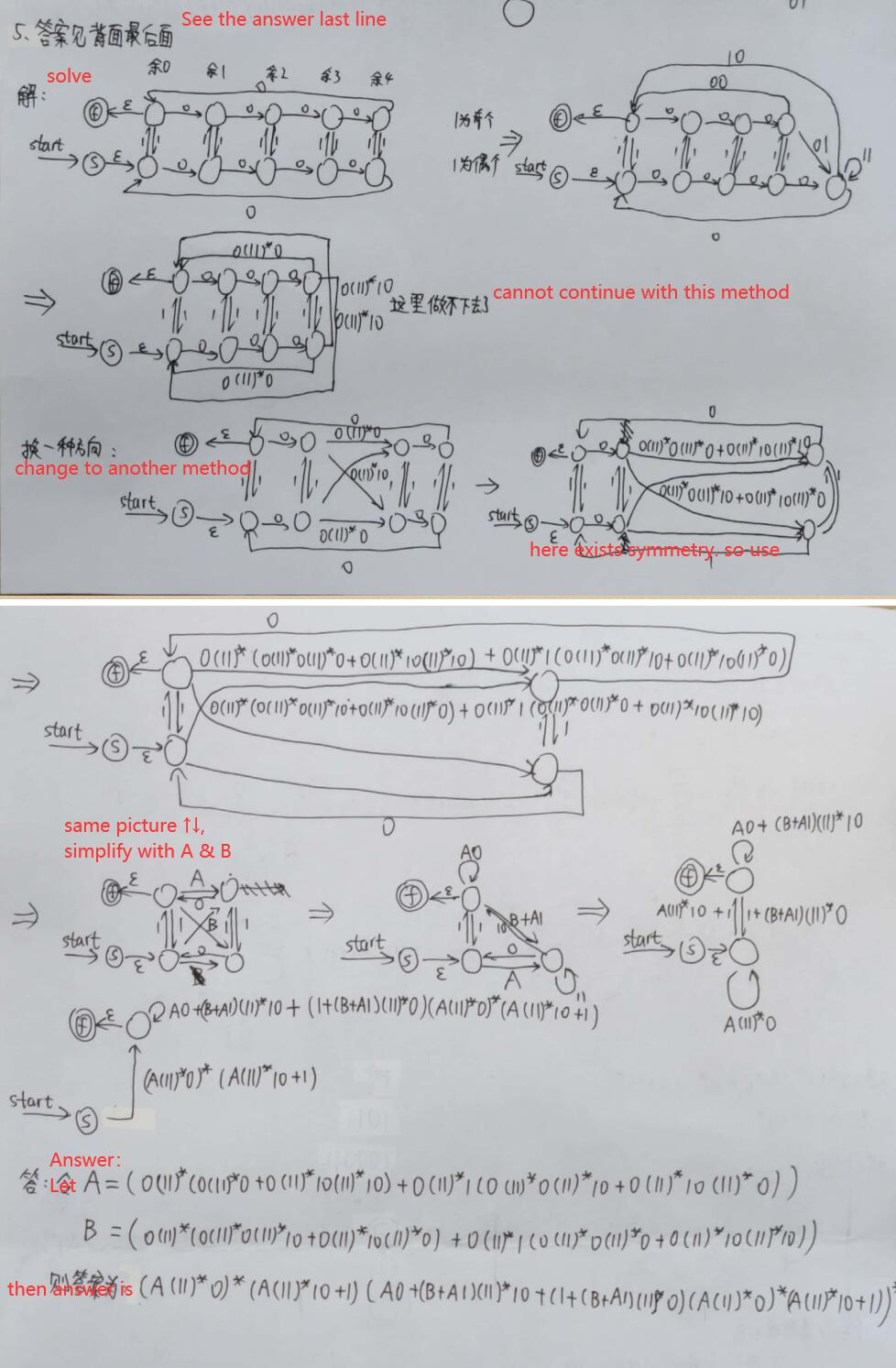##### What is the regular expression for the set of strings of 0's and 1's whose number of 0's is divisible by 5 and whose number of 1's is even??

What is the regular expression for the set of strings of 0's and 1's whose number of 0's is divisible by 5 and whose number of 1's is even??

shivani
25 Jul 2017 08:42 am
• The given problem can be solved by just cartesian product of two regular expressions.
• r=r1Xr2
• where r1 is (1*01*01*01*01*01*)* // regular expression for divisibility by 5.
• where r2 is (0*10*10*)* // regular expression for divisibility by 2.
• therefore,final regular expression  (r) is  (1*01*01*01*01*01*)*X(0*10*10*)*
Vaibhav Gupta
24 Jul 2017 08:05 pm

Sorry ma'am but i couldn't get it.

I am having some doubts...

Just consider the case where r1 is 000100 and r2 is 01010, now r will be 00010001010.

But r is not satisfying the given condition of the problem i.e. number of 0's is divisible by 5 and number of 1's is even as in this case there are eight 0's and three 1's..

I don't know whether my doubt is relevent or not?

Rahul
24 Jul 2017 01:47 pm

Its very Lengthy question ,i can give you a way how to slove

->Draw DFA of D1-> divisible by n(0)'s 5

->Draw DFA of d2->n(A)=even

Do cross of D1 and d2 you find 10 state and select  appropriate final state

From DFA find Regular expression

Vaibhav Gupta
24 Jul 2017 08:08 pm

Ok Sir..

Thank You..

Ran Duan
30 Mar 2021 05:47 pm

I have tried it. Show off for reference.

Please point out the weak points! Thanks!Parth Sharma
24 Jul 2017 10:48 pm

Shivani i hope u r doing some mistake bcz what if i take 5 zeros and a 1 from r1 and  2 one from r2

For ex

100000 from r1 and 11 from r2

Finally we get 10000011 which has odd no. Of zeros

Parth Sharma
24 Jul 2017 10:52 pm

This can be solved by ANDing of 2 dfa or catesian product of 2 dfa

shivani
25 Jul 2017 08:43 am

sorry mistake in writing , i meant cartesian product instead of concatenation.
edit done.